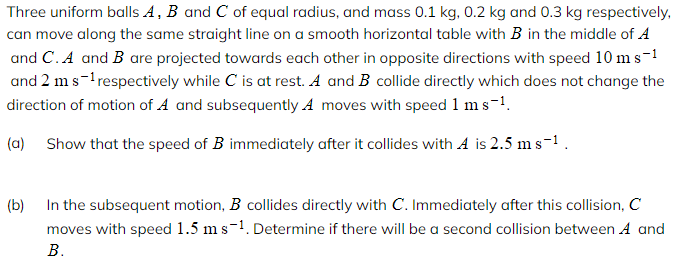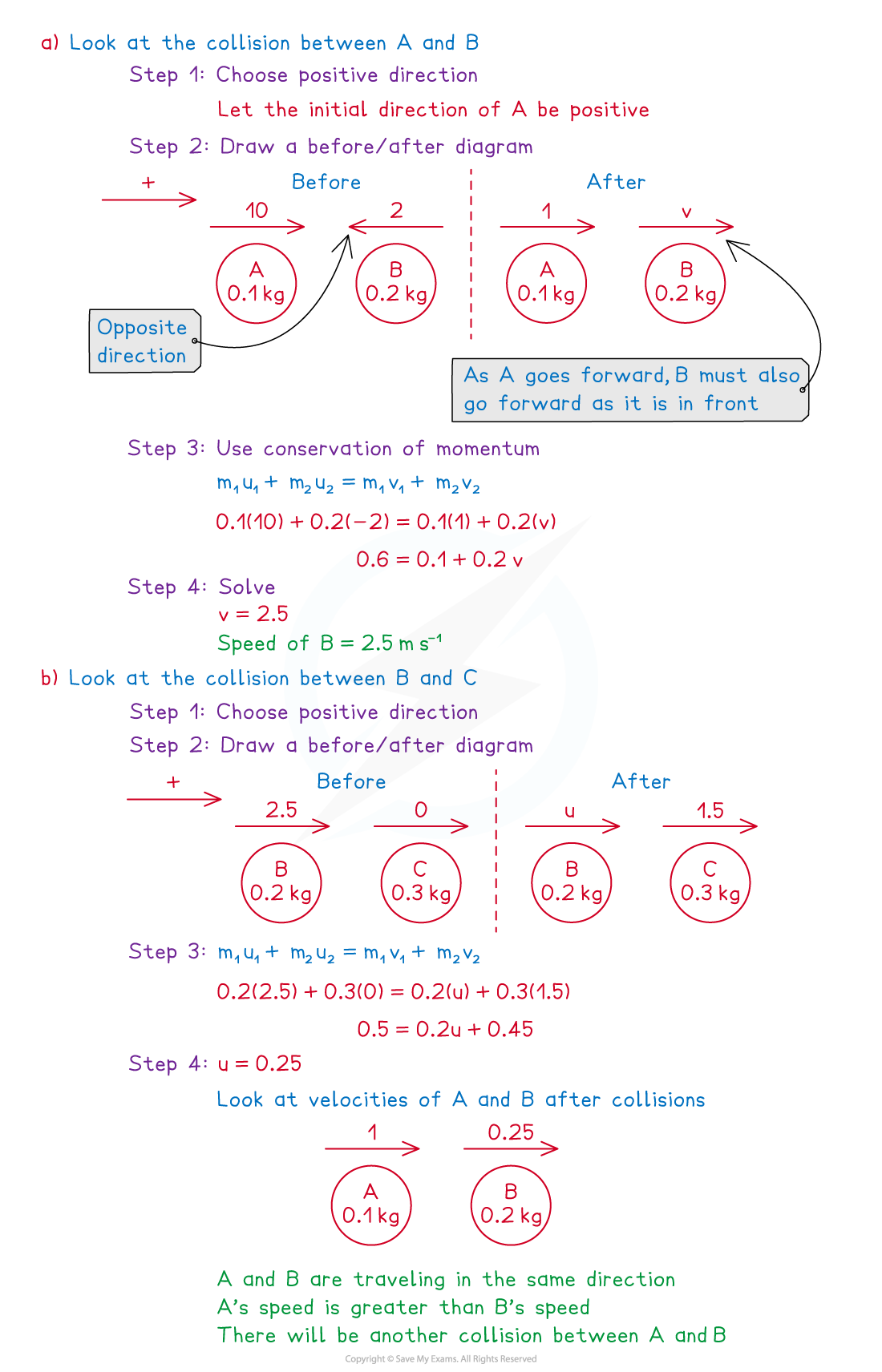# CIE A Level Maths: Mechanics复习笔记5.1.3 Collisions - Multiple Collisions

### Collisions - Multiple Collisions

#### Can there be multiple collisions?

• After two objects collide it is possible that one (or both) of them collides with something else such as
• third object
• wall (perpendicular to the motion)
• Deal with each collision separately and use the steps for direct collisions

#### Can there be a second collision between the original two objects?

• Let A, B and C be three objects travelling in the same straight line and suppose A and B collide directly and subsequently B and C collide directly
• After the collisions between A and B and B and C there will be a second collision between A and B if:
• One is stationary and the other is travelling towards it
• Both are travelling in opposite directions towards each other
• Both are travelling in the same direction and the one in front is slower than the one behind
• The process is similar if object C is a wall
• After B collides with the wall its direction will be reversed so it will be travelling towards A
• B will collide with A again if its velocity in that direction is greater than the velocity of A in that direction
• To help you work out the speed of B after hitting the wall you will be given extra information such as the change in momentum of B or loss in kinetic energy

#### Worked Example#### Exam Tip

• These questions can be difficult to visualise in your head so draw simple diagrams to show each collision.
• Use common sense, think how many possible (or impossible) ways there are for objects to move after the first collision. You will often have to consider the speed of one or more objects to decide if a second or third collision is possible.
• Questions can ask you to find the loss in kinetic energy so be sure to find the total kinetic energy before a collision and compare it to the total kinetic energy after a collision.
• These questions can involve lots of algebra, negatives and inequalities so do not rush them as you might make a silly mistake which can affect subsequent parts.